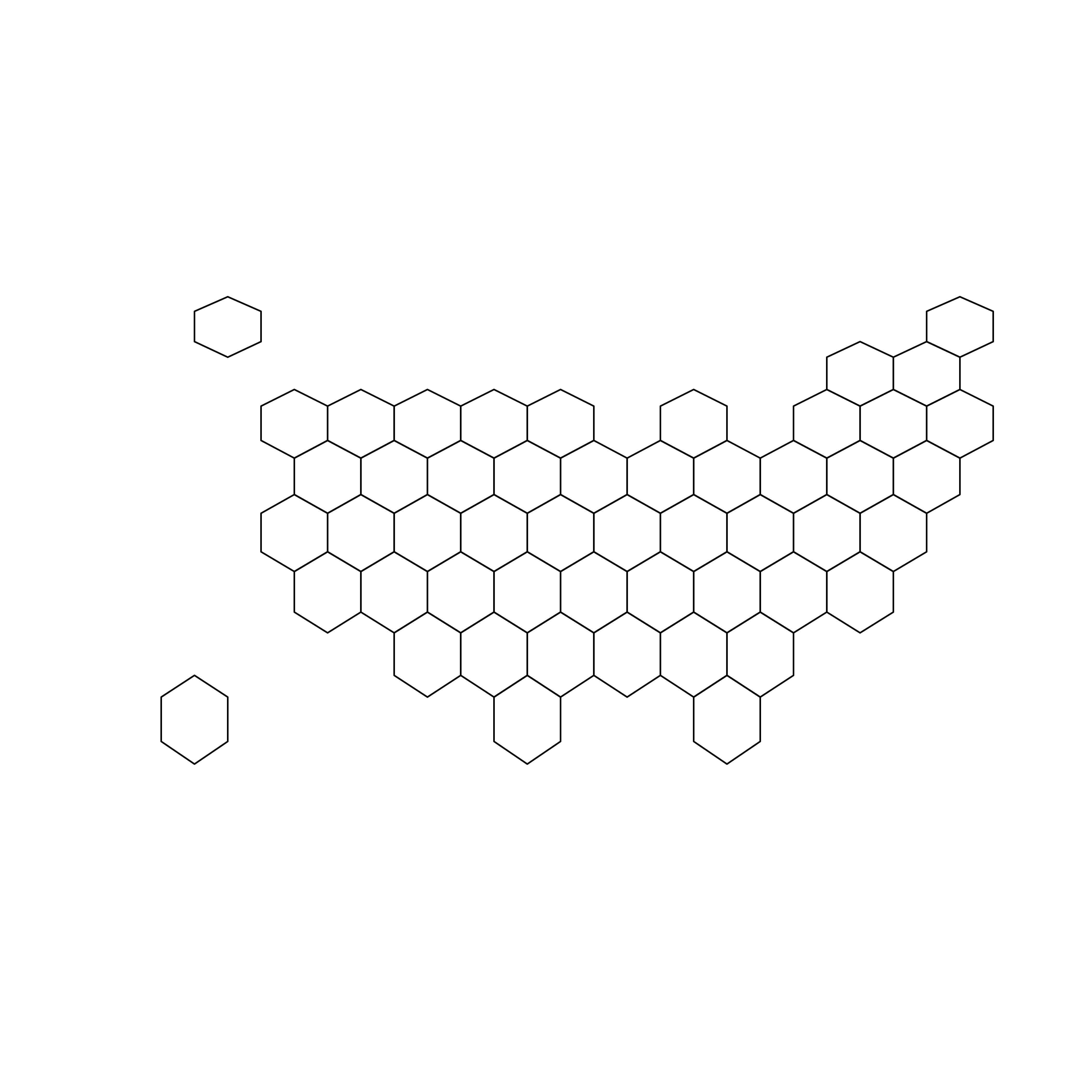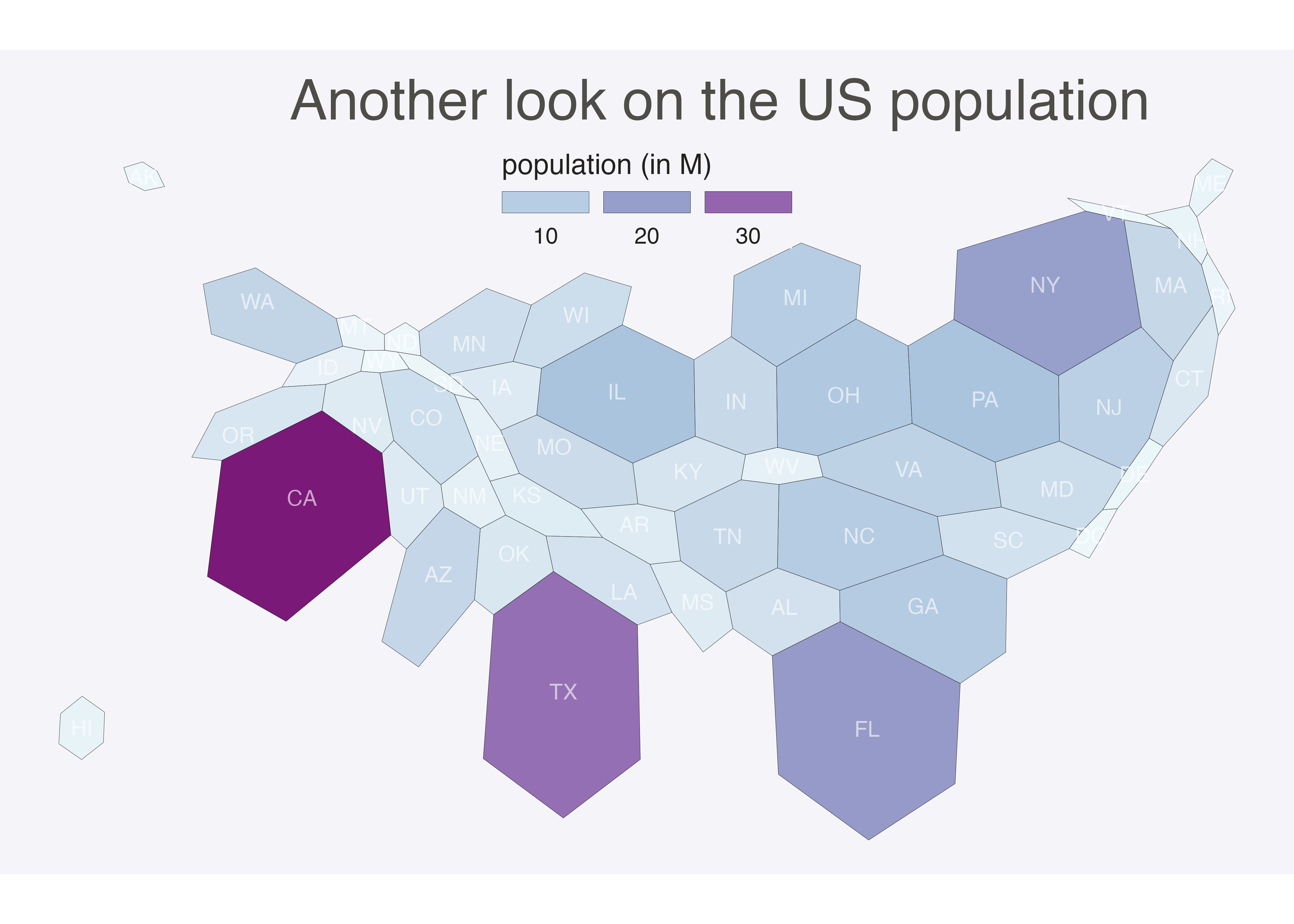# Basic Cartogram with R

This post describes how to apply the cartogram method to a hexbin map. Each region is represented as a hexagon which size is distorted according to a numeric variable. It uses the `cartogram` package.

# Basic hexbin map

The first step is to build a basic hexbin map of the US. Note that the gallery dedicates a whole section to this kind of map.

Hexagones boundaries are provided here. You have to download it at the `geojson` format and load it in R thanks to the `geojson_read()` function. You get a geospatial object that you can plot using the `plot()` function. This is widely explained in the background map section of the gallery.``````# library
library(tidyverse)
library(geojsonio)
library(RColorBrewer)
library(rgdal)

# Hexagones boundaries at geojson format were found here, and stored on my github https://team.carto.com/u/andrew/tables/andrew.us_states_hexgrid/public/map.

spdf <- geojson_read("https://raw.githubusercontent.com/holtzy/R-graph-gallery/master/DATA/us_states_hexgrid.geojson.json",  what = "sp")

# Bit of reformating
spdf@data = spdf@data %>%

# Show it
plot(spdf)``````

# Distort hexagone size with `cartogram`

The geospatial object has a data slot: an attached data frame that provides several information for each region. It is called `spdf@data` here.

We need to add a new column to this data frame. This column will provide the population per state, available at `.csv` format here.

We can thus use the `cartogram` library to distort the size of each state (=hexagon), proportionally to this column. The new geospatial object we get can be drawn with the same `plot` function.``````# Library
library(cartogram)

# Load the population per states (source: https://www.census.gov/data/tables/2017/demo/popest/nation-total.html)
pop\$pop <- pop\$pop / 1000000

# merge both
spdf@data <- spdf@data %>% left_join(., pop, by=c("google_name"="state"))

# Compute the cartogram, using this population information
cartogram <- cartogram(spdf, 'pop')

# First look!
plot(cartogram)``````

# Cartogram and choroplethTo get a satisfying result, let’s:

• color hexagones according to their population
• add state name. Label position is computed thanks to the `gCentroid()` function.
``````# Library
library(broom) # for the tidy function
library(rgeos) # for the gcentroid function

# tidy data to be drawn by ggplot2 (broom library of the tidyverse)
carto_fortified <- tidy(cartogram, region = "google_name")
carto_fortified <- carto_fortified %>%

# Calculate the position of state labels
centers <- cbind.data.frame(data.frame(gCentroid(cartogram, byid=TRUE), id=cartogram@data\$iso3166_2))

# plot
ggplot() +
geom_polygon(data = carto_fortified, aes(fill = pop, x = long, y = lat, group = group) , size=0.05, alpha=0.9, color="black") +
colours=brewer.pal(7,"BuPu"), name="population (in M)",
guide=guide_legend( keyheight = unit(3, units = "mm"),
keywidth=unit(12, units = "mm"),
title.position = 'top',
label.position = "bottom")
) +
geom_text(data=centers, aes(x=x, y=y, label=id), color="white", size=3, alpha=0.6) +
theme_void() +
ggtitle( "Another look on the US population" ) +

theme(
legend.position = c(0.5, 0.9),
legend.direction = "horizontal",
text = element_text(color = "#22211d"),
plot.background = element_rect(fill = "#f5f5f9", color = NA),
panel.background = element_rect(fill = "#f5f5f9", color = NA),
legend.background = element_rect(fill = "#f5f5f9", color = NA),
plot.title = element_text(size= 22, hjust=0.5, color = "#4e4d47", margin = margin(b = -0.1, t = 0.4, l = 2, unit = "cm")),
) +
coord_map()``````

Related chart types

## Contact

This document is a work by Yan Holtz. Any feedback is highly encouraged. You can fill an issue on Github, drop me a message on Twitter, or send an email pasting yan.holtz.data with gmail.com.